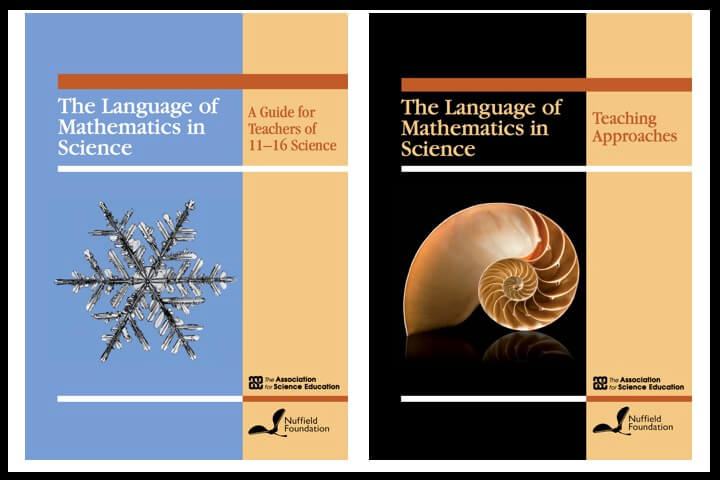Two useful reports that show interconnections between mathematics and science. A good read for all science and mathematics teachers.

The Language of Mathematics in Science: Guide

There are chapters on the following in this 132 page report:

1. Collecting data
2. Doing calculations and representing values
3. Choosing how to represent data
4. Drawing charts and graphs
5. Working with proportionality and ratio
6. Dealing with variability
7. Looking for relationships: line graphs
8. Looking for relationships: batches and scatter graphs
9. Scientific models and mathematical equations
10. Mathematics and the real world

The aim of this book is to enable teachers, publishers, awarding bodies and others to achieve a common understanding of important terms and techniques related to the use of mathematics in the science curriculum for pupils aged 11–16. The main part of the book consists of ten chapters, each focusing on a particular aspect of scientific activity, such as data collection, analysis, looking for relationships, presenting data, using scientific models, and so on.

The book provides:

• an overview of relevant ideas in secondary school mathematics and where they are used in science
• explanations of key mathematical terms along with a glossary giving definitions
• guidance about good practice in applying mathematical ideas in science.

The Language of Mathematics in Science: Teaching Approaches

There are Commentaries and Teacher Accounts in these areas

1. Cross-curricular approaches to graph drawing
3. Using a literacy approach to interpreting graphs
4. Introducing terms used to describe data types
5. Joint mathematics and science day to teach equations and graphs
6. The vocabulary of graphs – an example of departmental collaboration
7. Molar calculations in chemistry
8. Interpreting graphs

The aim of this book is to provide teachers, and others with an interest in science education, with authentic accounts of classroom practice describing the teaching of mathematics in science lessons to pupils aged 11–16. The main part of the book consists of eight teacher accounts of classroom activities designed to promote the use of some particular aspect of mathematics as part of learning science. Each account is accompanied by a narrative that sets the scene, provides a commentary on key points, and suggests a number of prompts to the reader to promote further reflection.

In this book you will find:

• examples of collaboration between mathematics and science teachers
• science learning activities designed to address specific mathematical ideas
• responses of individual pupils and groups to lesson activities